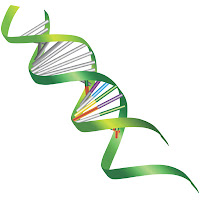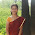## Saturday, April 19, 2014

### Genetic Algorithm I: Elements

This post is the first section on the implementation of Genetic algorithms in Scala: Basic components
Introduction
This post introduces the basic concepts behind Genetic Algorithms with an implementation in Scala. Scala is a type checked, object oriented and functional programming language built on top of Java Virtual Machine. We can leverage Scala's lambdas, partial functions and closures to implement the computational workflow of evolutionary  algorithm.
The second part of the implementation of genetic algorithm in Scala introduces the concept and implementation of genetic operations (cross-over, mutation and selection) on a population of chromosomes. Genetic Algorithms II: Operators
The 3rd and final post on genetic algorithms, explores the application of genetic algorithm as a solver or optimizer Genetic Algorithms III: Solver

Note: For the sake of readability of the implementation of algorithms, all non-essential code such as error checking, comments, exception, validation of class and method arguments, scoping qualifiers or import is omitted

TheoryGenetic Algorithms have been invented by John Holland in the 1970s and derived their properties from the theory of evolution of Darwin. A living organism consists of cells which are composed of identical chromosomes. Chromosomes are strings of DNA and serves as a model for the whole organism. A chromosome consist of genes that are blocks of DNA and encodes a specific protein. Recombination (or crossover) is the first stage of reproduction. Genes from parents generate the whole new chromosome (offspring) that can be mutated. During mutation, one or more elements, also known are individuals of the DNA strand or chromosome is changed. This changes are mainly caused by errors in copying genes from parents. The success of the organism in its life measures its fitness.
In computer science, Genetic Algorithms are a way of solving problems by mimicking nature. They use the same combination of selection, recombination and mutation to evolve a set of candidates for resolving a given problem. The basic computational sequence is
1. Select the initial population (search space) as a set of chromosones which represent candidates for solving a problem.
2. Encode the chromosones into a vector of real values (continuous process)  X(x1,..,xn) or string of bits (00101110101111010111).
3. Evaluate or rank the chromosones using a fitness function.
4. Select the fittest chromosones (which meet the minimum fitness criteria) for reproduction.
5. Pair chromosones for cross-over. The process consists of applying a cross-over rate to interchange fragment or section of the "parent" chromosones from a random point in the encoded string or vector.
6. Mutate chromosomes by flipping one or more of their elements(bits) using a randomly generated index bounded by a mutation rate.
7. Iterate the reproduction cycle (steps 2 to 6) for the current population.
Each of the genetic operators (selection, cross-over and mutation) relies on a parameter:
• Selection rate is the random  threshold value to reduce the current population of chromosones according to their fitness
• Crossover rate is used to compute the index beyond with the elements or bits of two parents chromosones are exchange.
The following parents
10001001110010010 1010001001000011 will generate the following offsprings 10001001110010011 and 1010001001000010
• Mutation Rate is used to compute the index of the element(s) or bit(s) in a chromosome that is/are mutated (or flipped).10001001110010010 becomes 10001001110000010

Chromosomes
The first step is to implement the key components of a genetic algorithm The implementation has to be generic enough to support any kind of fitness functions and encoding scheme.
A chromosomes is composed of one or several genes, each representing a predicate, axiom, fact or a rule/policy.
A Chromosone class wraps a list of parameterized genes, code (genetic code) (line 1). The most important methods related to chromosomes are
• +- for the cross-over between two chromosomes (line 6)
• ^ for the mutation of each chromosome (line 11)
• /= for the normalization of the fitness value of each chromosome during the selection process (line 14)
This implementation uses the unfitness value of the chromosome for ranking, instead the usual fitness value. It is defined as 1- normalized_fitness (line 3).

  1 2 3 4 5 6 7 8 9 10 11 12 13 14 15 16 final class Chromosome[T <: Gene](val code: List[T]) { var unfitness: Double = 1000*(1.0 + Random.nextDouble) // cross-over def +- ( that: Chromosome[T], gIdx: GeneticIndices): (Chromosome[T], Chromosome[T])= {} // mutation def ^ (gIdx: GeneticIndices): Chromosome[T] = {} // normalization of fitness def /= (normalizeFactor: Double): Unit = {} ... } 

The class GeneticIndices converts the cross-over and mutation probability (or rate) into indices (or position) on the bits of the chromosome or gene the cross-over and mutation is applied. The parameter chOpIdx defines the index along the bit string of a chromosome and geneOpIdx the index along the bit string of a gene

case class GeneticIndices(chOpIdx: Int, geneOpIdx: Int)


The generic indices are generated from the cross-over or mutation rate (probability) by the method geneticIndices (line 1). The index of the bit for genetic operations on the chromosome is defined as chIdx (ratio over the length of the chromosome) (lines 5 - 8). Likewise, the index or position of the bit genetic operations on the genes is defined as gIdx (line 11)

  1 2 3 4 5 6 7 8 9 10 11 12 13 14 def geneticIndices(prob: Double): GeneticIndices = { var idx = (prob*chromosomeSize).floor.toInt val chIdx = if(idx == chromosomeSize) chromosomeSize-1 else idx idx = (prob*geneSize).floor.toInt val gIdx = if(idx == geneSize) geneSize-1 else idx GeneticIndices(chIdx, gIdx) } 

Genes
The class Gene wraps the genetic code associated to a predicate or a rule and takes four parameters:
• id: This is the identifier of the gene. It is usually the name of the variable represented by the gene (line 2).
• target: This is the target value or threshold to be converted or discretized into a bit string (line 3).
• op: This is the operator that is applied to the target value (line 4).
• discr: This is the discretization (or quantization) scheme that converts a numerical (real) value to an integer which is then converted into bits. It also supports the conversion of the bits string back to a numerical value.
The genes as subjected to the same genetic operations as the chromosomes: cross-over (line 8) and mutation (line 9). The conversion from bits string back to numerical value is implemented by the method deconde (line 11).

  1 2 3 4 5 6 7 8 9 10 11 12 class Gene( val id: String, target: Double, op: Operator)(implicit discr: Discretization) { val bits: Bitset def +- (that: Gene, gIdx: GeneticIndices): Gene = {} def ^ (gIdx: GeneticIndices): Gene = ^ (gIdx.geneOpIdx) def ^ (idx: Int): Gene = {} def decode(bits: BitSet): (Double, Operator) { } } 

Quantization
The class Discretization has two arguments
• A function, toInt, converts a real value to an integer (line 2)
• A reverse function toDouble converts the integer back to a real value (line 3)
The main benefit to encapsulate the two conversions into a single class is to reduce the risk of inconsistency and inaccuracy between these two conversions.

  1 2 3 4 5 6 7 8 9 10 11 12 13 class Discretization( toInt: Double => Int, toDouble: Int => Double) { def this(R: Int) = this( (x: Double) => (x*R).floor.toInt, (n: Int) => n/R ) } def decode(bits: BitSet): (Double, Operator) = (discr.toDouble(convert(geneBits.rValue, bits)), op(convert(geneBits.rOp, bits)) ) 

The instantiation of Gene (lines 5-6) converts a predicate into a bit string of type java.util.BitSet. The bit string is decoded by the decode method of the Gene companion object (lines 10 - 12).

Genetic population
The Population class takes two parameters:
• limit: This is the maximum size of the population (line 4)
• chromosomes: This is the pool of chromosomes defining the current population (line 5).
A reproduction cycle executes the following sequence of three genetic operators on a population:
select for the selection of the fittest chromosomes of the population (line 8)
+- for the pair-wise crossover of all the chromosomes (line 1)
^ for the mutation of each chromosome (line 12).

  1 2 3 4 5 6 7 8 9 10 11 12 13 14 type Pool[T <: Gene] = ArrayBuffer[Chromosome[T]] class Population[T <: Gene]( limit: Int, val chromosomes: Pool[T]) { // selection operator def select(score: Chromosome[T] => Unit, cutOff: Double) // cross-over operator def +- (xOver: Double) // mutation operator def ^ (mu: Double) // ... } 

This completes the first part of the implementation of genetic algorithms in Scala. The next post dives into the details in the implementation of the genetic operators.

References
- Tutorial Darell Whitley - Colorado State University
- Short Tutorial from University of California, Davis
- Genetic Algorithms in Search, Optimization & Machine Learning D. Goldberg Addison-Wesley
- Programming in Scala M. Odersky, L. Spoon, B. Venners - Artima 2010
- Scala for Machine Learning - Patrick Nicolas - Packt Publishing

#### 1 comment:

1.Great and useful article. Creating content regularly is very tough. Your points are motivated me to move on.

SEO Company in Chennai